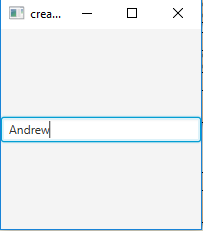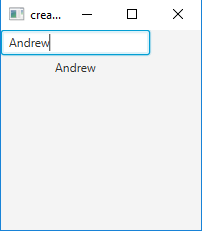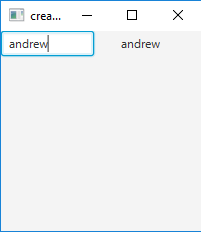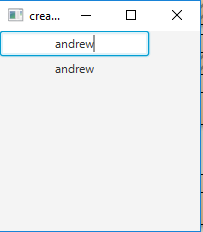Related Articles
JavaFX | TextField
• Last Updated : 31 Oct, 2019

TextField class is a part of JavaFX package. It is a component that allows the user to enter a line of unformatted text, it does not allow multi-line input it only allows the user to enter a single line of text. The text can then be used as per requirement.

Constructor of the TextField class :

1. TextField(): creates a new TextField with empty text content
2. TextField(String s): creates a new TextField with a initial text .

Commonly used methods:

method explanation
setPrefColumnCount(int v) Sets the value of the property prefColumnCount.
setOnAction(EventHandler value) Sets the value of the property onAction.
setAlignment(Pos v) Sets the value of the property alignment.
prefColumnCountProperty() The preferred number of text columns
onActionProperty() The action handler associated with this text field, or null if no action handler is assigned.
getPrefColumnCount() Gets the value of the property prefColumnCount.
getOnAction() Gets the value of the property onAction.
getAlignment() Gets the value of the property alignment.
getCharacters() Returns the character sequence backing the text field’s content.

Below programs illustrate the use of text field:

1. Java program to create a TextField and add it to stage: This program creates a TextField indicated by the name b. The TextField will be created inside a scene, which in turn will be hosted inside a stage (which is the top level JavaFX container). The function setTitle() is used to provide title to the stage. Then a Title-pane is created, on which addChildren() method is called to attach the TextField inside the scene, along with the resolution specified by (200, 200) in the code. Finally the show() method is called to display the final results.

 `// Java program to create a textfield and add it to stage ` `import` `javafx.application.Application; ` `import` `javafx.scene.Scene; ` `import` `javafx.scene.control.*; ` `import` `javafx.scene.layout.StackPane; ` `import` `javafx.stage.Stage; ` `public` `class` `Textfield ``extends` `Application { ` ` `  `    ``// launch the application ` `    ``public` `void` `start(Stage s) ` `    ``{ ` `        ``// set title for the stage ` `        ``s.setTitle(``"creating TextField"``); ` ` `  `        ``// create a textfield ` `        ``TextField b = ``new` `TextField(); ` ` `  `        ``// create a stack pane ` `        ``StackPane r = ``new` `StackPane(); ` ` `  `        ``// add textfield ` `        ``r.getChildren().add(b); ` ` `  `        ``// create a scene ` `        ``Scene sc = ``new` `Scene(r, ``200``, ``200``); ` ` `  `        ``// set the scene ` `        ``s.setScene(sc); ` ` `  `        ``s.show(); ` `    ``} ` ` `  `    ``public` `static` `void` `main(String args[]) ` `    ``{ ` `        ``// launch the application ` `        ``launch(args); ` `    ``} ` `} `

Output:2. Java program to create a TextField with an initial text and add an event handler: This program creates a TextField indicated by the name b. We will create a label which will display the Text when the enter key is pressed.we will create an event handler that will handle the event of the Text field and the event handler would be added to the Textfield using setOnAction() method. The TextField will be created inside a scene, which in turn will be hosted inside a stage (which is the top level JavaFX container). The function setTitle() is used to provide title to the stage. Then a Title-pane is created, on which addChildren() method is called to attach the TextField and a label inside the scene, along with the resolution specified by (200, 200) in the code. Finally, the show() method is called to display the final results.

 `// Java program to create a textfield and add a ` `// event handler to handle the event of textfield ` `import` `javafx.application.Application; ` `import` `javafx.scene.Scene; ` `import` `javafx.scene.control.*; ` `import` `javafx.scene.layout.*; ` `import` `javafx.event.ActionEvent; ` `import` `javafx.event.EventHandler; ` `import` `javafx.scene.control.Label; ` `import` `javafx.stage.Stage; ` `public` `class` `Textfield_1 ``extends` `Application { ` ` `  `    ``// launch the application ` `    ``public` `void` `start(Stage s) ` `    ``{ ` `        ``// set title for the stage ` `        ``s.setTitle(``"creating textfield"``); ` ` `  `        ``// create a textfield ` `        ``TextField b = ``new` `TextField(``"initial text"``); ` ` `  `        ``// create a tile pane ` `        ``TilePane r = ``new` `TilePane(); ` ` `  `        ``// create a label ` `        ``Label l = ``new` `Label(``"no text"``); ` ` `  `        ``// action event ` `        ``EventHandler event = ``new` `EventHandler() { ` `            ``public` `void` `handle(ActionEvent e) ` `            ``{ ` `                ``l.setText(b.getText()); ` `            ``} ` `        ``}; ` ` `  `        ``// when enter is pressed ` `        ``b.setOnAction(event); ` ` `  `        ``// add textfield ` `        ``r.getChildren().add(b); ` `        ``r.getChildren().add(l); ` ` `  `        ``// create a scene ` `        ``Scene sc = ``new` `Scene(r, ``200``, ``200``); ` ` `  `        ``// set the scene ` `        ``s.setScene(sc); ` ` `  `        ``s.show(); ` `    ``} ` ` `  `    ``public` `static` `void` `main(String args[]) ` `    ``{ ` `        ``// launch the application ` `        ``launch(args); ` `    ``} ` `} `

Output:3. Java program to create a textfield with an initial text and add an event handler: This program creates a TextField indicated by the name b. We will set an initial text by invoking its constructor with a string and also set the preferred column count using setPrefColumnCount() method. We will create a label which will display the Text when the enter key is pressed. We will create an event handler that will handle the event of the Text field and the event handler would be added to the Textfield using setOnAction() method. The TextField will be created inside a scene, which in turn will be hosted inside a stage (which is the top level JavaFX container). The function setTitle() is used to provide title to the stage. Then a Title-pane is created, on which addChildren() method is called to attach the TextField and a label inside the scene, along with the resolution specified by (200, 200) in the code. Finally, the show() method is called to display the final results.

 `// Java program to create a textfield with a initial text ` `// and preferred column count and add a event handler to ` `// handle the event of textfield ` `import` `javafx.application.Application; ` `import` `javafx.scene.Scene; ` `import` `javafx.scene.control.*; ` `import` `javafx.scene.layout.*; ` `import` `javafx.event.ActionEvent; ` `import` `javafx.event.EventHandler; ` `import` `javafx.scene.control.Label; ` `import` `javafx.stage.Stage; ` `public` `class` `TextField_2 ``extends` `Application { ` ` `  `    ``// launch the application ` `    ``public` `void` `start(Stage s) ` `    ``{ ` `        ``// set title for the stage ` `        ``s.setTitle(``"creating textfield"``); ` ` `  `        ``// create a textfield ` `        ``TextField b = ``new` `TextField(``"initial text"``); ` ` `  `        ``// set preffered column count ` `        ``b.setPrefColumnCount(``7``); ` ` `  `        ``// create a tile pane ` `        ``TilePane r = ``new` `TilePane(); ` ` `  `        ``// create a label ` `        ``Label l = ``new` `Label(``"no text"``); ` ` `  `        ``// action event ` `        ``EventHandler event = ``new` `EventHandler() { ` `            ``public` `void` `handle(ActionEvent e) ` `            ``{ ` `                ``l.setText(b.getText()); ` `            ``} ` `        ``}; ` ` `  `        ``// when enter is pressed ` `        ``b.setOnAction(event); ` ` `  `        ``// add textfield ` `        ``r.getChildren().add(b); ` `        ``r.getChildren().add(l); ` ` `  `        ``// create a scene ` `        ``Scene sc = ``new` `Scene(r, ``200``, ``200``); ` ` `  `        ``// set the scene ` `        ``s.setScene(sc); ` ` `  `        ``s.show(); ` `    ``} ` ` `  `    ``public` `static` `void` `main(String args[]) ` `    ``{ ` `        ``// launch the application ` `        ``launch(args); ` `    ``} ` `} `

Output:4. Java program to create a TextField with an initial text and center alignment of text and add an event handler: This program creates a TextField indicated by the name b.we will set an initial text by invoking its constructor with a string and also set the alignment using setAlignment() method.we will create a label which will display the Text when the enter key is pressed.we will create an event handler that will handle the event of the Text field and the event handler would be added to the Textfield using setOnAction() method. The TextField will be created inside a scene, which in turn will be hosted inside a stage (which is the top level JavaFX container). The function setTitle() is used to provide title to the stage. Then a Title-pane is created, on which addChildren() method is called to attach the TextField and a label inside the scene, along with the resolution specified by (200, 200) in the code. Finally, the show() method is called to display the final results.

 `// Java program to create a textfield with a initial text and center alignment of text ` `// and add a event handler to handle the event of textfield ` `import` `javafx.application.Application; ` `import` `javafx.scene.Scene; ` `import` `javafx.scene.control.*; ` `import` `javafx.scene.layout.*; ` `import` `javafx.event.ActionEvent; ` `import` `javafx.event.EventHandler; ` `import` `javafx.scene.control.Label; ` `import` `javafx.stage.Stage; ` `import` `javafx.geometry.*; ` `public` `class` `TextField_4 ``extends` `Application { ` ` `  `    ``// launch the application ` `    ``public` `void` `start(Stage s) ` `    ``{ ` `        ``// set title for the stage ` `        ``s.setTitle(``"creating textfield"``); ` ` `  `        ``// create a textfield ` `        ``TextField b = ``new` `TextField(``"initial text"``); ` ` `  `        ``// set alignment of text ` `        ``b.setAlignment(Pos.CENTER); ` ` `  `        ``// create a tile pane ` `        ``TilePane r = ``new` `TilePane(); ` ` `  `        ``// create a label ` `        ``Label l = ``new` `Label(``"no text"``); ` ` `  `        ``// action event ` `        ``EventHandler event = ``new` `EventHandler() { ` `            ``public` `void` `handle(ActionEvent e) ` `            ``{ ` `                ``l.setText(b.getText()); ` `            ``} ` `        ``}; ` ` `  `        ``// when enter is pressed ` `        ``b.setOnAction(event); ` ` `  `        ``// add textfield ` `        ``r.getChildren().add(b); ` `        ``r.getChildren().add(l); ` ` `  `        ``// create a scene ` `        ``Scene sc = ``new` `Scene(r, ``200``, ``200``); ` ` `  `        ``// set the scene ` `        ``s.setScene(sc); ` ` `  `        ``s.show(); ` `    ``} ` ` `  `    ``public` `static` `void` `main(String args[]) ` `    ``{ ` `        ``// launch the application ` `        ``launch(args); ` `    ``} ` `} `

Output:5. Note: The above programs might not run in an online IDE please use an offline compiler.

Attention reader! Don’t stop learning now. Get hold of all the important Java Foundation and Collections concepts with the Fundamentals of Java and Java Collections Course at a student-friendly price and become industry ready.

My Personal Notes arrow_drop_up
Recommended Articles
Page :